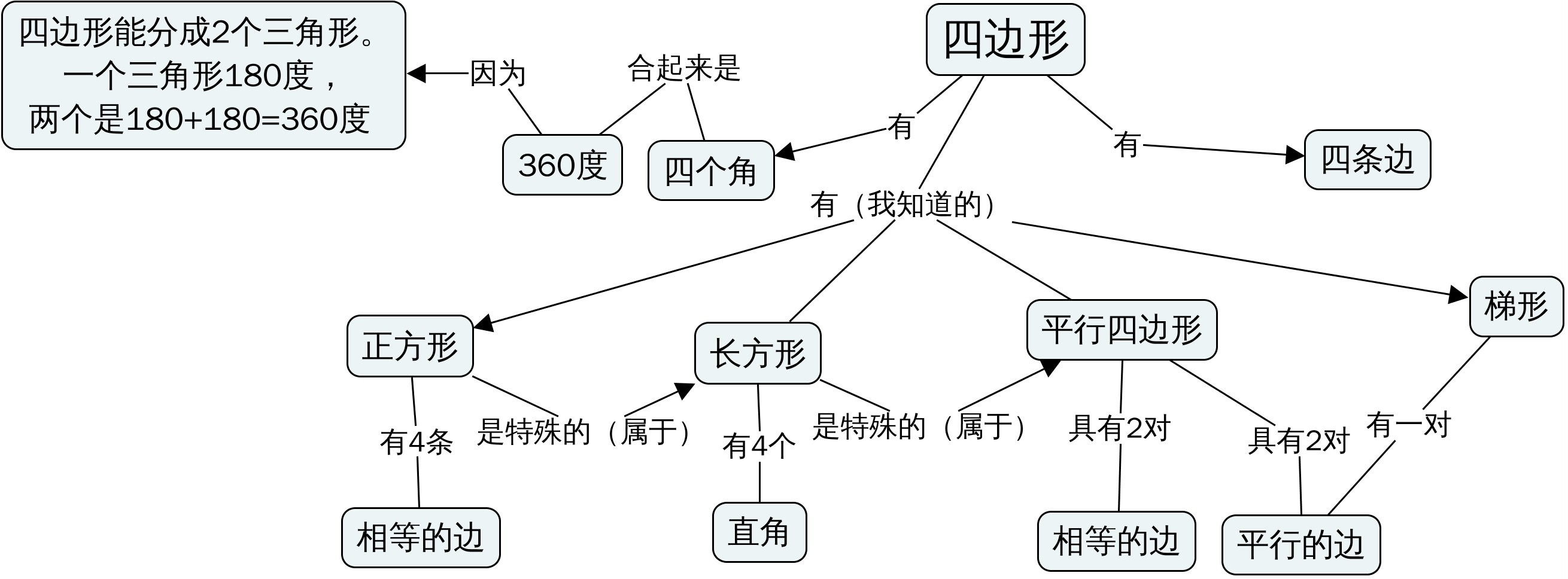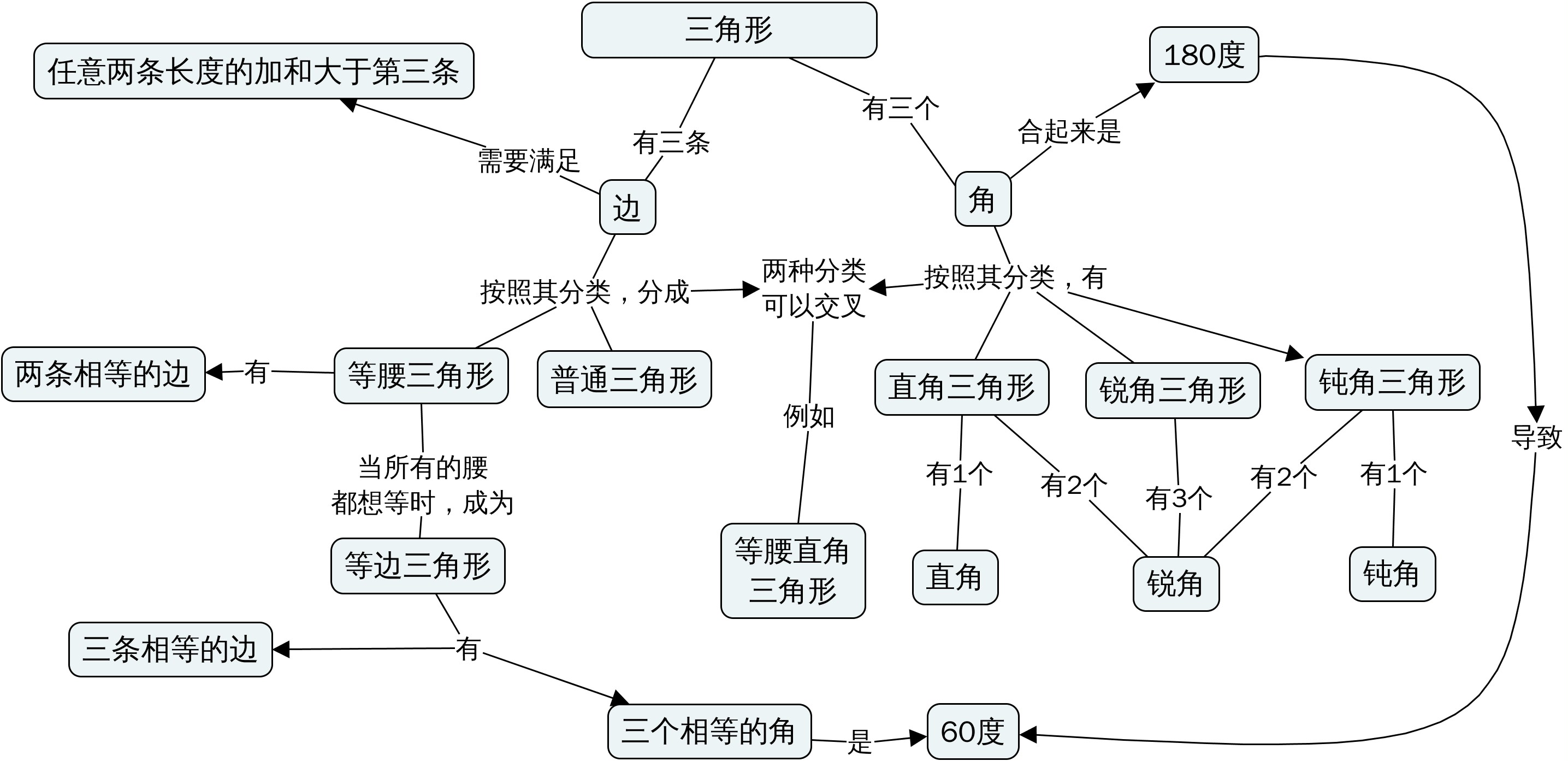## 加减乘除的计算规则## 理解型学习用于题海战术

\begin{align}
\left(56+13\right)\times 44 – 56\times\left(44-13\right)
\end{align}

\begin{align}
\left(56+13\right)\times 44 \times 56\times\left(44-13\right)
\end{align}

\begin{align}
13\times\left(56+13\right)+\left(44-13\right)\times 13
\end{align}

\begin{align}
13\times\left(56+13\right)= 13\times 56 \times 13
\end{align}

\begin{align}
13\times\left(56+13\right)=13\times 56\times 13\times 13
\end{align}

\begin{align}
13\times\left(56+13\right)+\left(44-13\right)\times 13 \\
=13\times 56 + 13\times 13+44\times 13 -13 \times 13 （乘法对加法的分配律） \\
=13\times 56 +44\times 13 + 13\times 13　-13 \times 13 （加法交换律） \\
=\left(13\times 56 +44\times 13\right) + \left(13\times 13　-13 \times 13\right) （加减法结合律） \\
=\left(13\times 56 +44\times 13\right) （算出来最后的减法） \\
=\left(56\times 13 +44\times 13\right) （乘法交换律） \\
=\left(56+44\right)\times 13 （乘法对加法的分配律） \\
=100\times 13 （算出来加法） \\
=1300　（算出来乘法）
\end{align}

## 退括号的机械式学习和理解型学习

1. 括号外面是加号的，里面是加减号的，展开以后里面的算符不变。
2. 括号外面是减号的，里面是加减号的，展开以后里面的算符变号。
3. 括号外面是加减号的，里面是乘除号的，展开以后里面的算符变号，但是先算乘除后加减。
4. 括号外面是乘号的，里面是乘除号的，展开以后里面的算符不变。
5. 括号外面是除号的，里面是乘除号的，展开以后里面的算符变号。
6. 括号外面是乘号的，里面是加减号的，展开以后里面的算符不变，乘号要用分配率乘到每一个上去。
7. 括号右边是除号的，里面是加减号的，展开以后里面的算符不变，除号要用分配率除到每一个上去。
8. 括号左边是除号的，里面是加减号的，没法打开括号。

## 为什么我非常讨厌“正方形是特殊的长方形”这句话